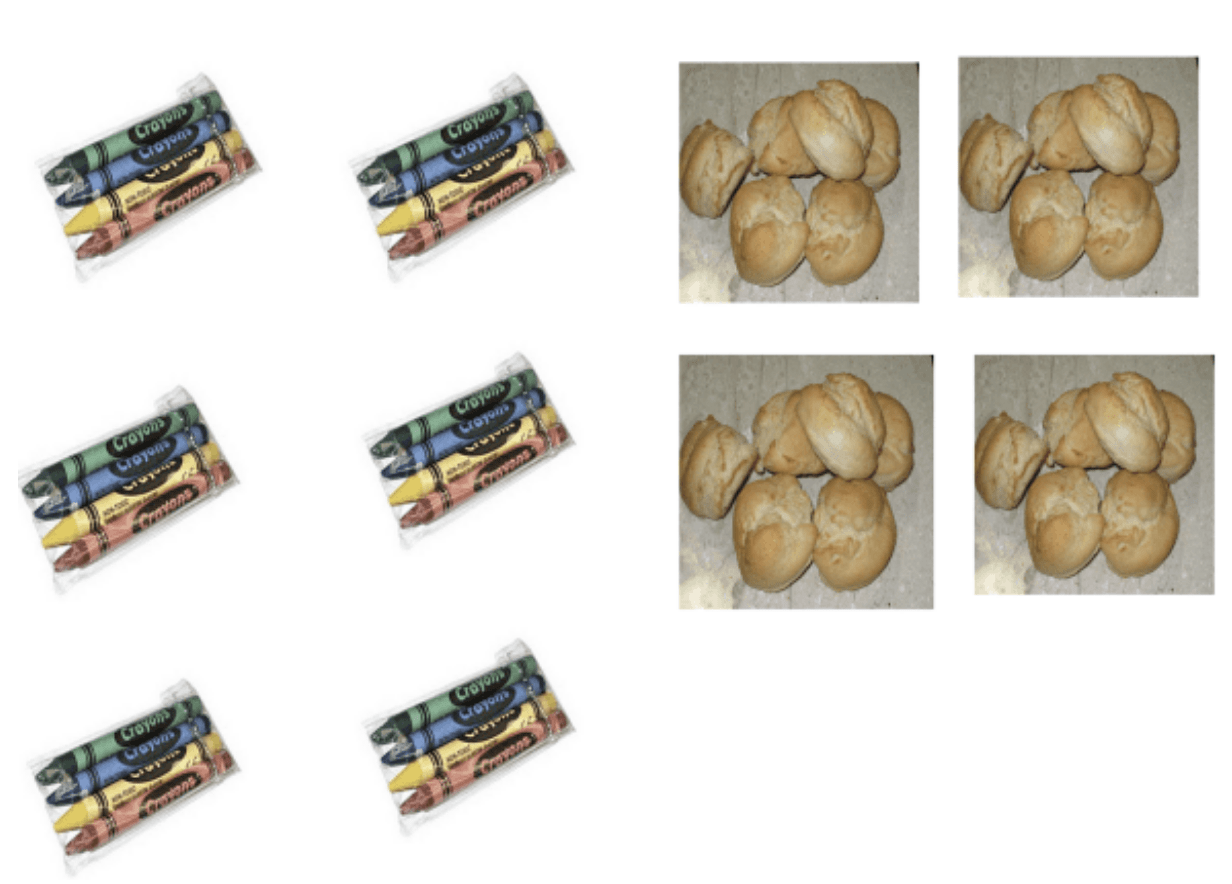# Early Multiplication

Classes 301 and 302 are ready to begin our next unit- Early Multiplication.  Our unit begins by  exploring multiplicative situations.  We’ll  start by looking at some photos of equal groups that we might encounter in life, such as egg cartons, boxes of markers, or multiple bags of marbles. The pictures are meant to generate a discussion of multiplication as equal groups, and how the order of the factors must reflect the reality of a problem’s context.

Many students are already aware of the commutative property of multiplication– that 4×6=6×4. Both of these equations total 24, however, each equation can represent very different situations. Here is an example:The crayons image is an example of 6 x 4, 6 boxes of crayons with 4 crayons in each,  or “6 groups of 4”. The bread rolls image depicts the equation 4 x 6, 4 groups of rolls, with 6 rolls in each, or “4 groups of 6”. This is a common convention of multiplication, that the first factor tells you how many groups, while the second factor tells you how many in the group.  We  have begun to use mathematical notation to describe the groups. Many students are even using the distributive property.

An example of the distributive property connected to the crayon image would be noticing that there are 3 groups of 4 on the left and 3 groups of 4 on the right. Therefore, (3×4) + (3 x 4) = 6 x 4

It’s important for multiplication to be taught in a context. Pictures are a natural way to introduce students to a foundational big ideas in multiplication. To really think multiplicatively, children have to think of the group as a unit.  Students build upon what they know in addition: 6 + 6 + 6 + 6 can also be thought of as 4 groups of 6.

The purpose of making groups, and groups of groups, is to find the total in an efficient way to find a total. When completing homework, you can support us in this by saying “groups of” rather than “times” as you talk about multiplication.  Over the next few weeks, you will begin to see your child using a range of strategies, such as:

• repeated addition  6 + 6 + 6 + 6=4 x 6 =24
• skip counting.    6, 12, 18, 24   in order to solve 6×4
• distributive property of multiplication –  Some examples: (3×4) + (3 x 4) = 6 x 4     OR    6×4= (2×4) + (2×4) + (2×4)
• commutative property of multiplication–  4×6=6×4 = 24

This series of lessons in this unit are meant to develop an understanding of the meaning of multiplication–  the emphasis is not on memorizing the basic multiplication facts. As students build a conceptual knowledge of multiplication, and develop a range of strategies for solving multiplicative situations, students will work to learn the facts.

THANK YOU FOR ALL YOUR DONATIONS TOWARDS OUR SALT CLAY RELIEF MAP PROJECT!

Upcoming Dates:

November 15th- Parent Teacher Conferences (1/2 day)

November 16th- BSI Pajama Day- Wear your pajamas to school! No slippers please.

November 22-23- No school- Happy Thanksgiving!

November 28th- Trip to College of Staten Island by bus (No chaperones needed- Thank you!)